# In Millikan's experiment, the charge on each drop of oil was measured in coulombs. Imagine the...

In Millikan's experiment, the charge on each drop of oil was measured in coulombs. Imagine the same experiment, but with charges measured in a fictitious unit called a zeet (Z).

 Drop Charge (Z) A 2.25×10−14 B 4.05×10−14 C 4.50×10−14 D 4.95×10−14 E 6.75×10−14

What is the charge on an electron in zeets?

We can determine the charge on the electron in zeets, by finding the greatest coom factor among the measured charges and their differences. The differences can be calculated by subtracting each of the values from the previous value,

B-A = 4.05×10−14-2.25×10−14 = 1.85×10−14

C-B =4.50×10−14 -4.05×10−14= 0.45×10−14

D-C = 4.95×10−14-4.50×10−14= 0.45×10−14

E-D = 6.75×10−14-4.95×10−14 = 1.80×10−14

The greatest common factor is 0.45×10−14, so the charge on the electron in zeets is 0.45×10−14zeets.

#### Earn Coin

Coins can be redeemed for fabulous gifts.

Similar Homework Help Questions
• ### In Millikan's experiment, the charge on each drop of oil was measured in coulombs. Imagine the...

In Millikan's experiment, the charge on each drop of oil was measured in coulombs. Imagine the same experiment, but with charges measured in a fictitious unit called a zeet (Z). Drop Charge (Z) A 1.44×10−14 B 2.88×10−14 C 3.24×10−14 D 3.60×10−14 E 5.04×10−14 What is the charge on an electron in zeets?

• ### In Millikan's experiment, the charge on each drop of oil was measured in coulombs. Imagine the...

In Millikan's experiment, the charge on each drop of oil was measured in coulombs. Imagine the same experiment, but with charges measured in a fictitious unit called a zeet (Z ). Drop Charge (Z ) A 7.80×10−15 B 1.82×10−14 C 2.08×10−14 D 2.34×10−14 E 3.38×10−14 What is the charge on an electron in zeets? Express your answer in zeets using two significant figures.

• ### Part C In Millikan's experiment, the charge on each drop of oil was measured in coulombs....

Part C In Millikan's experiment, the charge on each drop of oil was measured in coulombs. Imagine the same experiment, but with charges measured in a fictitious unit called a zeet ( Z ). Drop Charge ( Z ) A 3.42×10−14 B 5.70×10−14 C 6.27×10−14 D 6.84×10−14 E 9.12×10−14 What is the charge on an electron in zeets?

• ### Part C In Millikan's experiment, the charge on each drop of oil was measured in coulombs....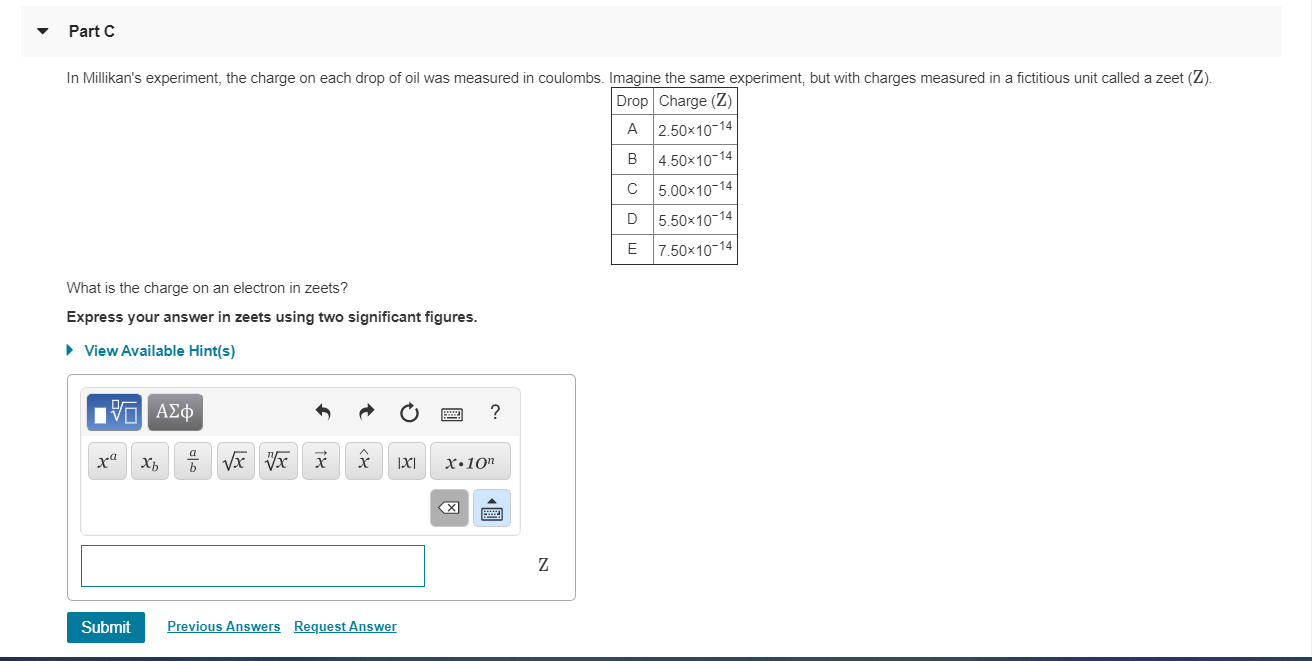Part C In Millikan's experiment, the charge on each drop of oil was measured in coulombs. Imagine the same experiment, but with charges measured in a fictitious unit called a zeet (Z). Drop Charge (Z) A 2.50x10-14 B 4.50x10-14 C 5.00x10-14 D 5.50*10-14 E 7.50*10-14 What is the charge on an electron in zeets? Express your answer in zeets using two significant figures. View Available Hint(s) IVO ACO x 6 8 x x x x x1 x 10" Z Submit...

• ### Milikans oil drop What is the charge on an electron in zeets?

In Millikan's experiment, the charge on each drop of oil was measured in coulombs. Imagine the same experiment, but with charges measured in a fictitious unit called azeet (z).Drop Charge ()A 1.70×10-14B 3.06×10-14C 3.40×10-14D 3.74×10-14E 5.10×10-14What is the charge on an electron in zeets?

• ### To analyze the experiment used to determine the properties of an electron. In 1909, Robert Millikan...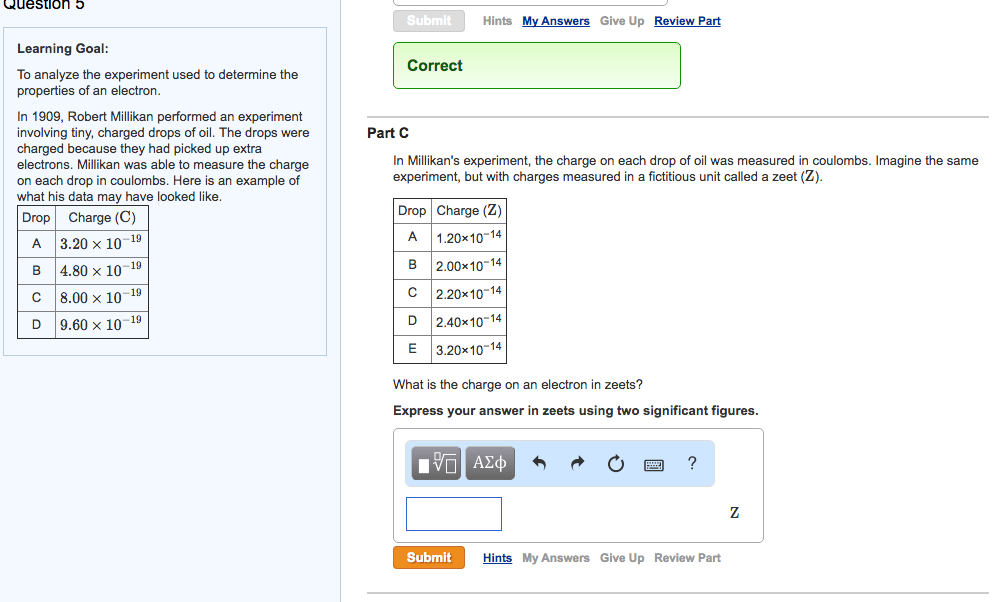To analyze the experiment used to determine the properties of an electron. In 1909, Robert Millikan performed an experiment involving tiny, charged drops of oil. The drops were charged because they had picked up extra electrons. Millikan was able to measure the charge on each drop in coulombs. Here is an example of what his data may have looked like. In Millikan's experiment, the charge on each drop of oil was measured in coulombs. Imagine the same experiment, but with...

• ### ecture Honework Chepter 02 Millikan's Oil-Drop Experiment 2 of 10 Leview Constants | Periodic Table Pi Aswers...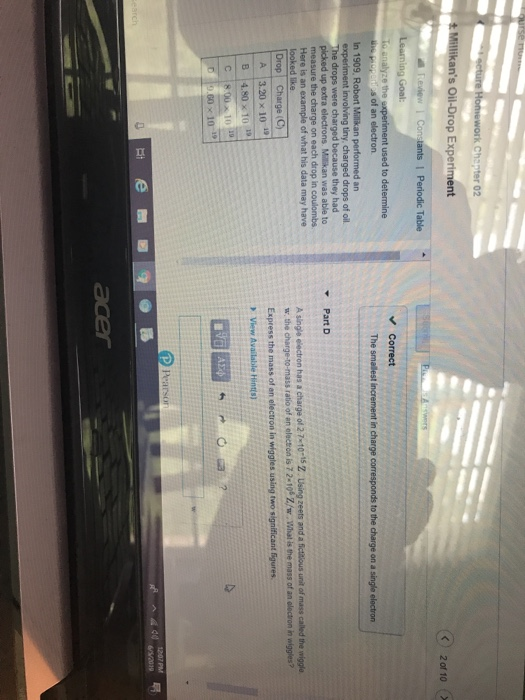ecture Honework Chepter 02 Millikan's Oil-Drop Experiment 2 of 10 Leview Constants | Periodic Table Pi Aswers Learning Goal: Correct To analyze the experiment used to determine he properios of an electron The smallest increment in charge corresponds to the charge on a single electron In 1909, Robert Millikan performed an experiment involving tiny, charged drops of oil. he drops were charged because they had picked up extra electrons. Milikan was able to measure the charge on each drop in...

• ### Millikan's oil drop experiment

In 1909 Robert Millikan was the first to find the charge of an electron in his now-famous oil drop experiment. In the experiment, tiny oil drops are sprayed into auniform electric field between a horizontal pair of oppositely charged plates. The drops are observed with a magnifying eyepiece, and the electric field is adjusted sothat the upward force on some negatively charged oil drops is just sufficient to balance the downward force of gravity. That is, when suspended, upward force...

• ### In Millikan's oil-drop experiment, one looks at a small oil drop held motionless between two plates....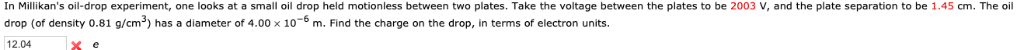In Millikan's oil-drop experiment, one looks at a small oil drop held motionless between two plates. Take the voltage between the plates to be 2003 V, and the plate separation to be 1.45 cm. The oil drop (of density 0.81 g/cm) has a diameter of 4.00 x 10m. Find the charge on the drop, in terms of electron units. x e 12.04 In Millikan's oil-drop experiment, one looks at a small oil drop held motionless between two plates. Take the...

• ### In Millikan's experiment, an oil drop of radius 2.012 µm and density 0.828 g/cm3 is suspended...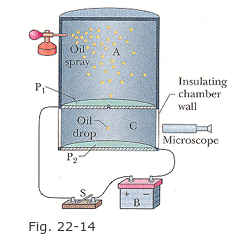In Millikan's experiment, an oil drop of radius 2.012 µm and density 0.828 g/cm3 is suspended in chamber C (Figure 22-14) when a downward-pointing electric field of 1.92* 10^5 N/C is applied. Find the charge on the drop, in terms of e. (Include the sign.) Thank youuuuu Oil spray Insulating chamber wall drop Microscope Fig. 22-14

Free Homework Help App